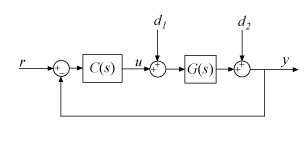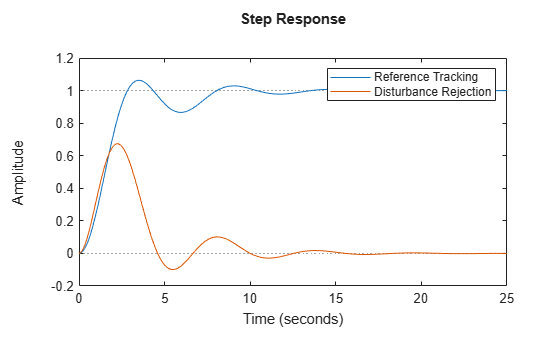# getPIDLoopResponse

Closed-loop and open-loop responses of systems with PID controllers

## Syntax

``response = getPIDLoopResponse(C,G,looptype)``

## Description

example

````response = getPIDLoopResponse(C,G,looptype)` returns a response of the control loop formed by the PID controller `C` and the plant `G`. The function returns the closed-loop, open-loop, controller action, or disturbance response that you specify with the `looptype` argument. The function assumes the following control architecture.When `C` is a `pid` or `pidstd` controller object (1-DOF controller):When `C` is a `pid2` or `pidstd2` controller object (2-DOF controller):```

## Examples

collapse all

Design a PI controller for a SISO plant and examine its performance in reference tracking and disturbance rejection. For reference tracking, use the `"closed-loop"` response. For rejection of a load disturbance, use `"input-disturbance"`.

```G = tf(1,[1 1 1]); C = pidtune(G,'PI'); Tref = getPIDLoopResponse(C,G,"closed-loop"); Tdist = getPIDLoopResponse(C,G,"input-disturbance"); step(Tref,Tdist) legend("Reference Tracking","Disturbance Rejection")```Validate the tuned controller by comparing the extracted responses to your design requirements for settling time and overshoot.

Design a two-degree-of-freedom (2-DOF) PID controller for a plant and examine its performance in reference tracking and disturbance rejection. For reference tracking, use the `"closed-loop"` response. For rejection of a load disturbance, use `"input-disturbance"`.

```G = tf(1,[1 0.5 0.1]); w0 = 1.5; C = pidtune(G,'PID2',w0); Tref = getPIDLoopResponse(C,G,"closed-loop"); Tdist = getPIDLoopResponse(C,G,"input-disturbance"); step(Tref,Tdist) legend("Reference Tracking","Disturbance Rejection")```## Input Arguments

collapse all

PID controller, specified as a PID controller object (`pid`, `pidstd`, `pid2`, or `pidstd2`).

Plant, specified as a SISO dynamic system model, such as a `tf`, `ss`, `zpk`, or `frd` model object. If `G` is a model with tunable or uncertain elements (such as a `genss` or `uss` model), then the function uses the current or nominal value of the model.

Loop response to return, specified as a string or character vector. The available loop responses are given in the following table.

Response1-DOF Controller2-DOF ControllerDescription
`"open-loop"`GC–GCyResponse of the open-loop controller-plant system. Use for frequency-domain design.
Use when your design specifications include robustness criteria such as open-loop gain margin and phase margin.
`"closed-loop"`

$\frac{GC}{1+GC}$ (from r to y)

$\frac{G{C}_{r}}{1-G{C}_{y}}$ (from r to y)

Closed-loop system response to a step change in setpoint. Use when your design specifications include setpoint tracking.
`"controller-effort"`

$\frac{C}{1+GC}$ (from r to u)

$\frac{{C}_{r}}{1-G{C}_{y}}$ (from r to u)

Closed-loop controller output response to a step change in setpoint. Use when your design is limited by practical constraints, such as controller saturation.
`"input-disturbance"`

$\frac{G}{1+GC}$ (from d1 to y)

$\frac{G}{1-G{C}_{y}}$ (from d1 to y)

Closed-loop system response to load disturbance (a step disturbance at the plant input). Use when your design specifications include input disturbance rejection.
`"output-disturbance"`

$\frac{1}{1+GC}$ (from d2 to y)

$\frac{1}{1-G{C}_{y}}$ (from d2 to y)

Closed-loop system response to a step disturbance at plant output. Use when you want to analyze sensitivity to modeling errors.

## Output Arguments

collapse all

Selected loop response, returned as a state-space (`ss`) or frequency-response data (`frd`) model. If `G` is an `frd` model, then `response` is also an `frd` model with the same frequencies as `G`. Otherwise, `response` is an `ss` model.

## Version History

Introduced in R2019a